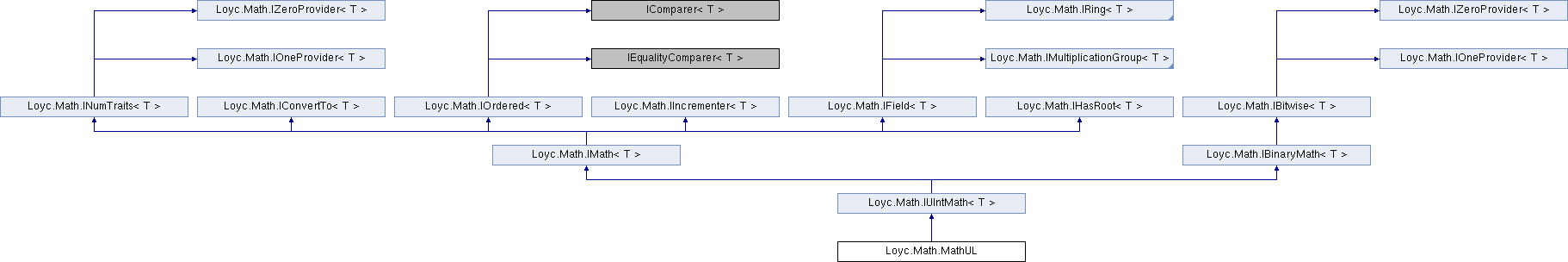Enhanced C# Language of your choice: library documentation

# Documentation moved to ecsharp.net

GitHub doesn't support HTTP redirects, so you'll be redirected in 3 seconds.

Loyc.Math.MathUL Struct Reference

Implements IUIntMath{T} for numbers of type System.UInt64. More...

Source file:
Inheritance diagram for Loyc.Math.MathUL:## Remarks

Implements IUIntMath{T} for numbers of type System.UInt64.

## Public static fields

static readonly MathUL Value = new MathUL()

## Properties

MinValue` [get]`

MaxValue` [get]`

Epsilon` [get]`

PositiveInfinity` [get]`

NegativeInfinity` [get]`

NaN` [get]`

bool IsSigned` [get]`

bool IsFloatingPoint` [get]`

bool IsInteger` [get]`

bool IsOrdered` [get]`

int SignificantBits` [get]`

int MaxIntPowerOf2` [get]`

ulong MaxInt` [get]`

long MinInt` [get]`

Zero` [get]`

One` [get]`Properties inherited from Loyc.Math.INumTraits< T >
MinValue` [get]`
Minimum value of this type above negative infinity. More...

MaxValue` [get]`
Maximum value of this type below infinity. More...

Epsilon` [get]`
Smallest representable positive value of T (1 for integer types). More...

PositiveInfinity` [get]`
Returns positive infinity, or MaxValue for types that cannot represent infinity. More...

NegativeInfinity` [get]`
Returns negative infinity, or throws NotSupportedException if T is unsigned. More...

NaN` [get]`
Not-a-number or null representation for this type. More...

bool IsSigned` [get]`
Returns true if T can represent negative values. More...

bool IsFloatingPoint` [get]`
Returns true if T is floating-point, meaning that it can represent very large and very small numbers, despite possibly limited precision. Returns false for fixed-point and integer-rational types. More...

bool IsInteger` [get]`
Returns true if the type represents only whole numbers. More...

bool IsOrdered` [get]`
Returns true for "normal" numbers, false for ones that aren't necessarily comparable (notably complex numbers). More...

int SignificantBits` [get]`
Returns the normal maximum number of significant (mantissa) bits for this type (not counting the sign bit), or int.MaxValue for unlimited-size types. More...

int MaxIntPowerOf2` [get]`
Returns the maximum power-of-two-minus-one that can be represented by this type, e.g. for Int32 it's 31, and for UInt32 it's 32. More...

ulong MaxInt` [get]`
Returns the maximum integer that this type can represent. More...

long MinInt` [get]`
Returns the minimum integer that this type can represent. More...Properties inherited from Loyc.Math.IZeroProvider< T >
Zero` [get]`
Returns the "zero" or additive identity of this type. More...Properties inherited from Loyc.Math.IOneProvider< T >
One` [get]`
Returns the "one" or identity value of this type. More...

## Public Member Functions

bool IsInfinity (T value)
Returns true if the given value is infinite. More...

bool IsNaN (T value)
Returns true if the given value is not a number (can only be true for floats). More...

Floor (T a)
Gets the closest integer equal to or lower than the specified number. More...

Ceiling (T a)
Gets the closest integer equal to or higher than the specified number. More...

From (uint t)

From (int t)

From (ulong t)

From (long t)

From (double t)

Clip (uint t)

Clip (ulong t)

Clip (int t)

Clip (long t)

Clip (double t)

bool IsLess (T a, T b)

bool IsLessOrEqual (T a, T b)

Abs (T a)

Max (T a, T b)

Min (T a, T b)

int Compare (T x, T y)

bool Equals (T x, T y)

int GetHashCode (T x)

Returns a + 1. More...

SubOne (T a)
Returns a - 1. More...

NextHigher (T a)
Returns the next representable number higher than a. More...

NextLower (T a)
Returns the next representable number lower than a. More...

Add (T a, T b, T c)

Sub (T a, T b)

Mul (T a, T b)

Div (T a, T b)

MulDiv (T a, T mul, T div)

Shl (T a, int amount)
Shifts 'a' left by the specified number of bits. More...

Shr (T a, int amount)
Shifts 'a' right by the specified number of bits. More...

Sqrt (T a)

Square (T a)

And (T a, T b)

Or (T a, T b)

Xor (T a, T b)

Not (T a)

int CountOnes (T a)
Returns the number of '1' bits in 'a'. More...

int Log2Floor (T a)
Returns the floor of the base-2 logarithm of x. e.g. 1024 -> 10, 1000 -> 9 More...

## Member Function Documentation

 T Loyc.Math.MathUL.AddOne ( T a )
inline

Returns a + 1.

Implements Loyc.Math.IIncrementer< T >.

 T Loyc.Math.MathUL.Ceiling ( T value )
inline

Gets the closest integer equal to or higher than the specified number.

For integer types, this has no effect.

Implements Loyc.Math.INumTraits< T >.

 int Loyc.Math.MathUL.CountOnes ( T a )
inline

Returns the number of '1' bits in 'a'.

Implements Loyc.Math.IBinaryMath< T >.

 T Loyc.Math.MathUL.Floor ( T value )
inline

Gets the closest integer equal to or lower than the specified number.

For integer types, this has no effect.

Implements Loyc.Math.INumTraits< T >.

 bool Loyc.Math.MathUL.IsInfinity ( T value )
inline

Returns true if the given value is infinite.

Types that do not have an infinity value always return false from this method.

Implements Loyc.Math.INumTraits< T >.

 bool Loyc.Math.MathUL.IsNaN ( T value )
inline

Returns true if the given value is not a number (can only be true for floats).

Implements Loyc.Math.INumTraits< T >.

 int Loyc.Math.MathUL.Log2Floor ( T a )
inline

Returns the floor of the base-2 logarithm of x. e.g. 1024 -> 10, 1000 -> 9

The return value is int.MinValue for an input of zero (for which the logarithm is technically undefined.)

Implements Loyc.Math.IBinaryMath< T >.

 T Loyc.Math.MathUL.NextHigher ( T a )
inline

Returns the next representable number higher than a.

Implements Loyc.Math.IIncrementer< T >.

 T Loyc.Math.MathUL.NextLower ( T a )
inline

Returns the next representable number lower than a.

Implements Loyc.Math.IIncrementer< T >.

 T Loyc.Math.MathUL.Shl ( T a, int amount )
inline

Shifts 'a' left by the specified number of bits.

A shift amount A negative shift amount produces undefined results

Implements Loyc.Math.IBinaryMath< T >.

 T Loyc.Math.MathUL.Shr ( T a, int amount )
inline

Shifts 'a' right by the specified number of bits.

Implements Loyc.Math.IBinaryMath< T >.

 T Loyc.Math.MathUL.SubOne ( T a )
inline

Returns a - 1.

Implements Loyc.Math.IIncrementer< T >.